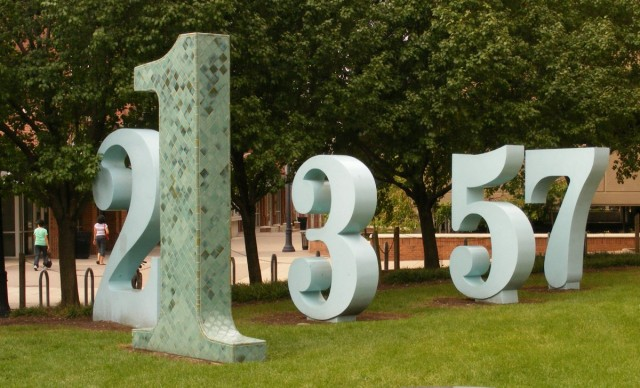# Kind of a Big Deal: Mathematician Proves Weak Version of Twin Prime ConjectureThe twin prime conjecture states that there are an infinite number of twin primes — prime numbers where one prime is two greater than the other. For example, 1 and 3 are twin primes, so are 101 and 103, 881 and 883, and so on. UPDATE: As pointed out in the comments, 1 is not a prime! You can learn more about that little fact right here. This conjecture has never been proven, but Yitang Zhang believes he has proven there are infinite primes paired with other primes no greater than 70 million more than themselves. Here’s why that’s kind of a big deal.

Zhang hasn’t proven an infinite number of true twin primes where one prime is two greater than the other, but he’s proven that there are paired primes separated by other values up to 70 million. Instead of proving the twin prime conjecture stating there are an infinite number of primes where p and p+2 are both prime, he has proven an infinite number of p and p + less than 70 million.

70 million and 2 are very different numbers, but Zhang’s work shows that there’s a limit to the distance between an infinite number of prime numbers, and that’s never been proven before. Also, the 70 million limit seems huge, but considering the new largest-known prime number is 17 million digits long, it’s really not that big in terms of the numbers mathematicians are dealing with.

Mathematicians have known for centuries that there are an infinite number of prime numbers, but the jury is still out on twin prime conjecture. Prime numbers tend to get further apart from each other the higher you go. There’s not a set pattern for it, but if you chart out known primes, you get something that looks almost like a loose spiral.

Zhang started working on the proof last summer when he had the idea. Since making it public, other mathematicians have been checking out Zhang’s work, and so far it holds up. The proof is currently under review, and if it passes muster it will be published in The Annals of Mathematics.

(via Phys Org, image via renoir_girl)

Relevant to your interests

Have a tip we should know? [email protected]

### Follow The Mary Sue:Glen is a comedian, writer, husband, and father. He won his third-grade science fair and is a former preschool science teacher, which is a real job.